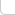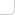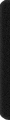# 某島

… : "…アッカリ～ン . .. . " .. .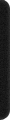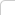June 21, 2012

## 主席樹學習筆記 Lv. 1

。。總之由於原名字太長了，而且 “主席” 兩個字念起來冷艷高貴，以後全部稱之為主席樹好了。。。

• 線段樹的每個結點，保存的是這個區間含有的數字的個數。
• 主席樹的每個結點，也就是每顆線段樹的大小和形態也是一樣的，也就是主席樹之間可以相互進行加減運算。。

• 主席樹是一種離線結構。。（必須預先知道所有數字的範圍。。這在一些應用中會成為障礙。。。
• 存在 MLE 問題。。（如果按照定義裡面的方法去寫，對每個結點都需要開一整可線段樹，至少都是 O(n2) 級別的空間。。

——————————————————

const int N = 50009, M = 10009, NN = 2500009;

int l[NN], r[NN], c[NN], total;

PII A[N+M]; int B[N+M], Q[M];
int S[N], C[N], Null;
int n, m, An, Tn;
.. .


l[], r[], c[], total 分別是左孩子下標，右孩子下標，該區間的數字個數和當前已分配的結點數。
（線段樹的區間可以在遞歸的時候實時計算，可以不予保存。。。

PII A[]; int B[]; A、B 用於離散化。。。 B 記錄相應數字的 Rank 值。
int Q[]; 記錄詢問。。。

int S[], C[], Null; 是主席樹下標。。

An 是 A 數組的長度，由初始 n 個數字和修改後的數字決定，
Tn 是所有出現的不同數字的個數，用於建立線段樹，由對 A 數組去重後得到。

#define lx l[x]
#define rx r[x]
#define ly l[y]
#define ry r[y]
#define cx c[x]
#define cy c[y]

#define mid ((ll+rr)>>1)
#define lc lx, ll, mid
#define rc rx, mid+1, rr

void Build(int &x, int ll, int rr){
x = ++total; if (ll < rr) Build(lc), Build(rc);
}

int Insert(int y, int p, int d){

int x = ++total, root = x;

c[x] = c[y] + d; int ll = 0, rr = Tn;

while (ll < rr){
if (p <= mid){
lx = ++total, rx = ry;
x = lx, y = ly, rr = mid;
}
else {
lx = ly, rx = ++total;
x = rx, y = ry, ll = mid + 1;
}
c[x] = c[y] + d;
}

return root;
}


。。。沒有修改的鏈仍然指向 y 的相對應部分。複雜度為樹高。。。。

。。。下面是 int main(); 函數代碼了。。。完整程序移步這裡。。。。

int main(){

#ifndef ONLINE_JUDGE
freopen("in.txt", "r", stdin);
//freopen("out.txt", "w", stdout);
#endif

#define key first
#define id second

RD(n, m); REP(i, n) A[i] = MP(RD(), i);

An = n; char cmd; REP(i, m){
RC(cmd); if(cmd == 'Q') RD(Q[i], Q[i], Q[i]);
else RD(Q[i]), Q[i] = 0, A[An++] = MP(RD(), An);
}

sort(A, A + An), B[A.id] = Tn = 0;
FOR(i, 1, An){
if(A[i].key != A[i-1].key) A[++Tn].key = A[i].key;
B[A[i].id] = Tn;
}

Build(Null, 0, Tn); REP_1(i, n) C[i] = Null;

S = Null; REP(i, n){
S[i+1] = Insert(S[i], B[i], 1);
}

An = n;

REP(i, m) if (Q[i]){
OT(A[Query(Q[i], Q[i], Q[i])].key);
}else{
Modify(Q[i], B[Q[i]-1], -1);
Modify(Q[i], B[Q[i]-1] = B[An++], 1);
}
}


1. 由於每棵線段樹的大小形態都是一樣的，而且初始值全都是 0，那每個線段樹都初始化不是太浪費了？所以一開始只要建一棵空樹即可。

事實上由於當一個結點的 c[] 結果為 0 時。。無論它向左還是向右遞歸下去結果都會是0 。。。
所以初始時甚至不是建立空樹。。而是只需要一個空結點即可。。。（如何實現這層。。。

2. 仍然沒有將 Lazy Evalutaion 的理念做到完美。。。這題中的 Lazy Evaluation 體現在兩個方面。。
主席樹的單鏈修改和主席樹與主席樹之間的運算。。

主席樹與主席樹之間的運算如果全部做的話一次是 O(n)。。。但是因為詢問時只需要計算一條到葉子的路徑。。。
。。。上面的代碼中，這種運算是通過 Struct Pack; 結構體現的。。。一種多顆主席樹結點的打包。。
。。全部在同一層的同一個位置。。向同一個方向遞歸下降。。一個 Pack; 的值為該結構內所有結點值的和。。

。。現在實現的不足之處：

• 無法很好的處理減運算。。因此這裡才還要保存了 S[] 前綴和，讓 C[] 逐個逐個修改。。。
。。。然後每次回答詢問的時候還要一併帶上 S[] 。。Orz。（雖然說有助於預處理。。
• 對計算過後結果無法有效利用。。（換句話說沒有體現出主席式 Memorization 的一面。。。
• 對單個鏈的插入操作也不是要即時處理的。。（。。如何用數據結構把所有操作先 Hold 下來 。。?。。這樣的好處是有一些操作對結果沒有影響是可以忽略。。另外把一堆操作放在一起當做一個操作處理的話有時也能得到 Bonus 。。。
3. Fotile 大神說如果加上垃圾回收的話，可以去掉一個 log…… ym

（另外正一個誤。。。加上回收並不能去掉一個 log 。。。。